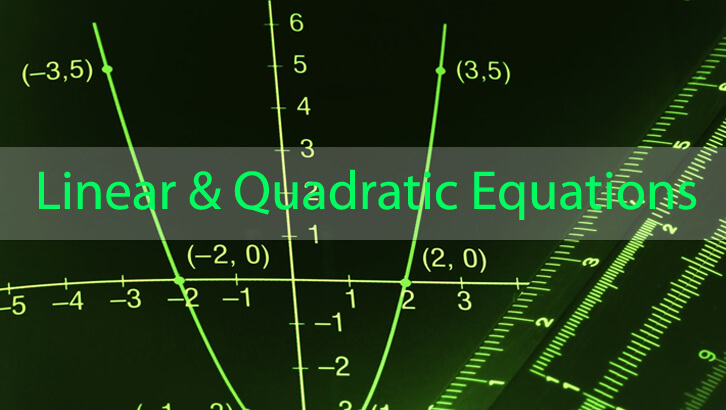# CAT Linear Equations

Another question from the book Quantitative Aptitude for CAT .

Question
1.       x + |y| = 8, |x| + y = 6.How many pairs of x, y satisfy these two equations?
A.      2
B.      4
C.      0

D.      1

We start with the knowledge that the modulus of a number can never be negative, though the number itself may be negative.
The first equation is a pair of lines defined by the equations
y = 8 – x        ——- (i) {when y is positive}
y = x – 8        ——- (ii) {when y is negative}
With the condition that x 8 (because if x becomes more than 8, |y| will be forced to be negative, which is not allowed)
The second equation is a pair of lines defined by the equations:
y = 6 – x        ——- (iii) {when x is positive}
y = 6 + x        ——- (iv) {when x is negative}
with the condition that y cannot be greater than 6, because if y > 6, |x| will have to be negative.
On checking for the slopes, you will see that lines (i) and (iii) are parallel. Also (ii) and (iv) are parallel (same slope).
Lines (i) and (iv) will intersect, but only for x = 1; which is not possible as equation (iv) holds good only when x is negative
Lines (ii) and (iii) do intersect within the given constraints. We get x = 7, y = -1. This satisfies both equations.
Only one solution is possible for this system of equations.

## One comment

1.savant says:

that was nice. sir, i’d like to know as to when the cat should be taken? early or late? when did you take it last year?